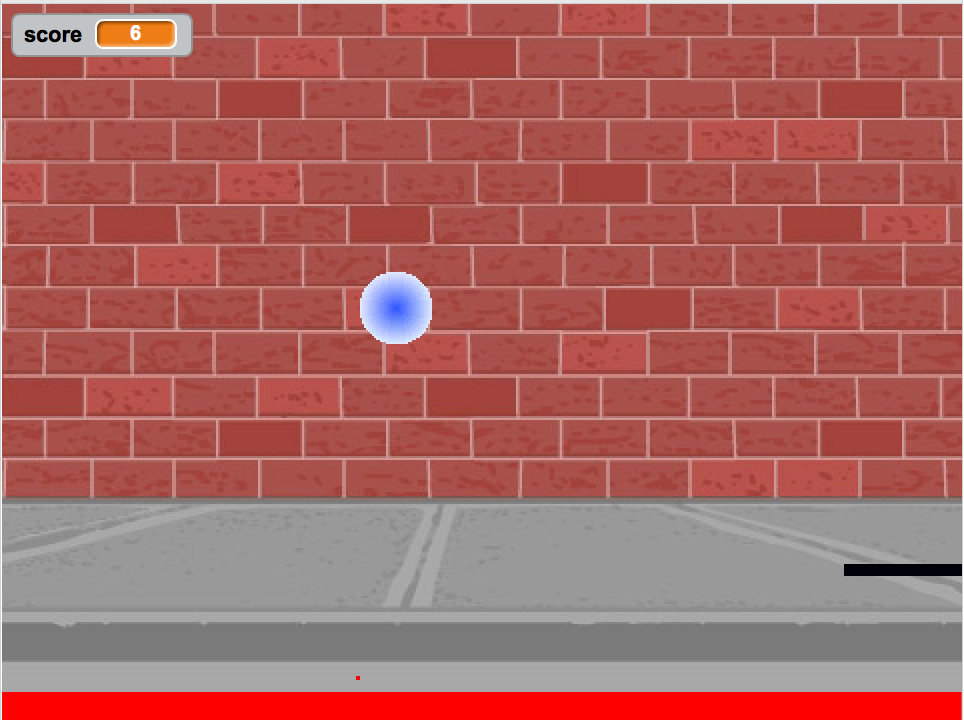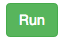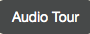# Compute with Numbers¶

We won’t be using lots of math in this eBook, so don’t worry if math isn’t your favorite thing. You will run and modify programs that work with words, turtles (virtual ones), and images as well as numbers. The only math that you need to know is addition, subtraction, multiplication, and division.

Computers were first used for calculations. You may be used to doing calculations with a calculator, but calculations are often easier if you can name the numbers you are working with. When you name a number, or the result of a calculation, you are creating a variable. A variable is a name associated with computer memory that can hold a value and that value can change or vary. One example of a variable is the score in a game. The score starts off at 0 and increases as you play the game.Figure 2: A pong game in Scratch with a score shown in the upper left.¶

One thing that you might want to calculate is a Body Mass Index. Body Mass Index (BMI) is a measure of body fat that is useful in screening for health issues. Generally speaking:

• A BMI of 18.5 or less is considered underweight.

• A BMI between 18.5 to 24.9 is considered healthy.

• A BMI between 25-29.9 is considered overweight.

• A BMI of 30 or over is considered obese.

To calculate a BMI, you need the height in inches and the weight in pounds. You square the height (multiply the height in inches by itself), then divide the weight in pounds by the squared-height. BMI is defined in terms of meters and kilograms, so to convert from pounds and inches multiply by 703.

In the box below is a computer program that calculates and prints the BMI for someone 60 inches tall (5 feet) and 110 pounds.

Press thebutton below to make the computer execute these steps. The output from this program will be displayed to the right of the program.

Pres thebutton below to bring up an “Audio Tour” that explains this program, line-by-line.

You can only use the Save and Load buttons if you are logged in. The Save button will save the current program and the Load button will load a saved program.

Change the weight (in inches) and height (in pounds) in the program above, and press the Run button to calculate a new BMI.